## Cardinality

at 9:20 p.m. on 19. May, 2013

It's time to make a post about MATHS.

Most people who know some mathematics know that there are infinitely many natural (whole) numbers. It's easy to see — you can always add one to a number and you get a brand new one. Clearly this also goes for many other sets: there are infinitely many even numbers, odd numbers, rational numbers (fractions) and real numbers (decimals). But one of these sets is not like the others — it is bigger than the rest!

Most people (unless they have inside knowledge) will think this is either obviously true or obviously false, and they will both be wrong! The first person will think that there are obviously more natural numbers than evens, more rational numbers than natural numbers, and more real numbers than rational numbers (since every fraction can be written as a possibly repeating decimal, but there are irrational numbers, like.) The second will think that they are all just infinite, and therefore the same size — if you add something to infinity, you still have infinity.

The first person is right, to a point. However in mathematics once you get to dealing with infinite sets we need to pin down what we mean by "size", and the concept we landed on is "cardinality." The upshot is just that two sets are the same size if the elements of each set can be paired off with each other, so that all elements of set A have one and only one corresponding element in set B, and vice versa.

Thus there are as many natural numbers as even numbers, because we can pair each numberwith the even number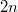. It's easy to see that there is one, and only one,for each, and one, and only onefor every even number — since an even number is a number in the form: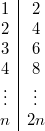What we've done is exhibited a bijection between the set of natural numbers,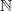, and the set of evens,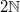. A set that is in bijection with the natural numbers is called countable. The link shows how it is possible to prove that the set of rational numbers is countable.

So, up until now the second person was correct, but no longer. Cantor proved in 1891 that there are more reals than there are naturals — that the set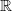of reals is uncountable.

The proof is quite simple. Supposeis countable, and we will find a contradiction, so what we supposed is impossible, andmust be of a different cardinality than. (And, since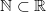, it is clearly bigger!)

To be countable, there must be a bijection — a function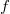fromtopairing off the elements with each other. We can illustrate such a function as follows: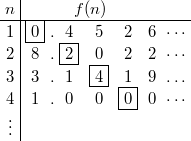Now we have to imagine that this table goes on forever down and right, since there are infinitely manys, and each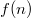can be infinitely long if it is irrational, but that's OK. This table just illustrates what part of one possible potential bijection would look like, and we are about to argue about any candidate — it's important to remember that whateverwe try, this argument will still apply, whether we try to modify it slightly, or pick a completely new one. It also doesn't matter that we only showed numbers between zero and ten.

Suppose we had indeed paired off everything, so that every real had its, then I will show how to find a new real number,. The idea is that we will show thatdoes not appear anywhere in the table, and hencewill not be a bijection (if it were, then every possible real, including ones we cook up in fanciful ways, must be listed.) Then, we will have a contradiction, meaning thatis not countable.

The contradiction follows from looking at the diagonal of thes. So, look at the first decimal digit of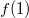— above it is 0. If it's zero, then the first digit ofwill be one, and otherwise it will be zero. So for the above table,starts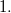. Then, look at the second digit of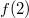. Do the same, sonow starts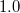. Continuing in this way, we get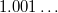, and theth digit ofisif theth digit ofis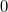, otherwise it is.

Now, ifwere indeed a bijection, as we want it to be, thismust be listed on the table somewhere — every real number is. But it is quite obvious thatdoes not appear, because it is different fromat position 1,at position 2, and so on — it is different fromat position!

And that is the contradiction. Any possible bijection — one-to-one pairing — of the natural numbers with the reals has this problem, so no such pairing can exist. Hence there really are more real numbers than there are natural numbers.

Now, some people will be entirely convinced by this while others might have objections. The first common objection says, "why couldn't we just add this new number to the list?" But remember, the argument above works with any possible listing, so just adding a number (or infinitely many!) to the list won't help; we can run the same procedure and find a missing number still.

The second common objection is that the notion of cardinality must somehow not be fit for purpose. After all, if it produces such weird results, why should we accept it as the mathematical notion of "size"? Well, first of all, even if we rejected cardinality as the correct definition of size, we'd still be left with the fact that we can't pair off the natural numbers with the reals, and if that's not because there are more reals than naturals, why is it? Second, it turns out that this concept is much more robust than any other notion of size when applied to infinite collections of things and so weird as it may be, cardinality is the best tool for the job!

Related tags: cantor cardinality diagonalisation maths reals set-theory